# Mathematical Physics

## Homework Help & Tutoring

We offer an array of different online Mathematical Physics tutors, all of whom are advanced in their fields and highly qualified to instruct you.Send your subject help request Submit your homework problem, or a general tutoring request.
Get quotes from qualified tutors Receive a response from one of our tutors as soon as possible, sometimes within minutes!
Mathematical Physics Tutors Available Now
48 tutors available
Mathematical Physics Homework Library
68 total solutions
See what our students are saying
Can you help me with my homework in less than 24 hours?
Can you help me with my exam/quiz/test?
How much will it cost?
What kind of payments do you accept?

# Mathematical Physics

Mathematical physics is the powerful tool that physicists and chemists use in their everyday research to predict the real life systems using abstract mathematical models. Using the equations of mathematical physics you can calculate an enormous amount of different systems – starting from a guitar string and ending with the radioactive activity of a specific element! Both of these real world systems are explained with partial differential equations.

In this article, this is the easiest example that exists in mathematical physics –  the derivation of the string equation, and on this example you will be able to notice the basic considerations of mathematical physics.

So, the string, as shown on the picture, will be a mathematical model.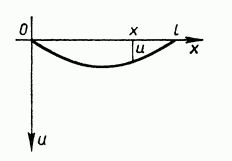First of all, we need to list the major approximations – we neglect width and height of the string, to be able to concentrate on length.  In addition, we will say that the string is absolutely flexible, which means that there is no resistance to any external force that bends the string.

So, as you see on the picture, we have a string with a length l. We say that there are tension forces T in the x direction and that the ends of the string are fixed.

To finish mathematical modeling we say that every point of the string moves in a direction orthogonal to the x axis; these oscillations are small and that string is homogeneous.

Since the oscillations are small the law of the oscillation can be given as a function of 2 variables: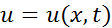, where u, as shown on the picture is the distance of a point on the string at coordinate x at time t from the x axis.

Now, we use the formula for an arc length to show, that at the time t: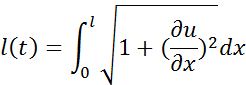We already stated that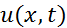is small. If the string is smooth enough, the derivative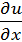is small as well. Hence, when we square this derivative, it will have a value with a higher order of infinitesimal. We will assume that these oscillations are so small that we neglect the derivative. Hence, the length of a string is: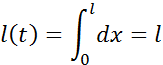That simply means that including small oscillations of the string, we consider the length to be constant. From the same considerations, any given part of a string will have constant length.

From the given assumptions about the characteristics of oscillations, we can get the next conclusions: since the length of the string is constant, the tension at any given point will be constant and be equal to T. Then we can see that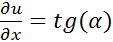, where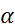is the angle between tangent and a string in point x and the time t (recall this formula from pre-calculus). So, we can derive: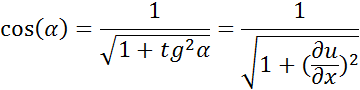However, from our assumption, we neglect the square of the derivative. So, at any given point of the string we have: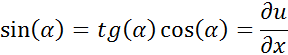From here we can start the derivation of the equation for the oscillation of a string. For this we take into consideration a small part of the string given by x and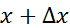. Since we assumed that the string is homogeneous the density is constant, and we will denote it as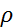. Then the mass of this part of the string will be equal to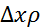. On two sides of this part of the string we have forces acting on it. They are equal in magnitude     (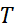), and opposite in direction.

The horizontal component of these forces is equal to zero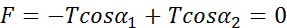The vertical component will be given as: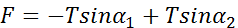Now we have: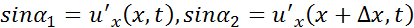which means that vertical component can be rewritten as: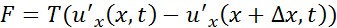From Lagrange theorem we can say that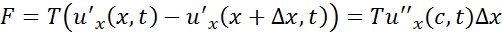where c is an arbitrary point that lies inside x and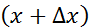. Now, applying Newton’s second law,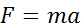,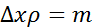, and remembering that acceleration is second derivative of distance by time, we can state that: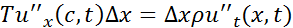If we cancel x and state that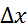is infinitesimal. Then c will be close to x and we will get: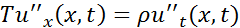Finally, if we denote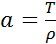, we obtain the final result for a string equation: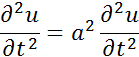This is a partial differential equation that completely describes the movement of a string. Amazingly, the same equation will be used if we want to know the thermal conductivity of a beam. There are multiple ways to solve these equations like separation of variables, or the Dirichlet method. If you would like to learn more about this topic, or if you have any other problem in mathematical physics, we will gladly help you!

You can read more about partial differential equations at MIT Open Courseware, and a useful set of notes on mathematical physics can be found here.

## College Mathematical Physics Homework Help

Since we have tutors in all Mathematical Physics related topics, we can provide a range of different services. Our online Mathematical Physics tutors will:

• Provide specific insight for homework assignments.
• Review broad conceptual ideas and chapters.
• Simplify complex topics into digestible pieces of information.
• Answer any Mathematical Physics related questions.
• Tailor instruction to fit your style of learning.

With these capabilities, our college Mathematical Physics tutors will give you the tools you need to gain a comprehensive knowledge of Mathematical Physics you can use in future courses.

## 24HourAnswers Online Mathematical Physics Tutors

Our tutors are just as dedicated to your success in class as you are, so they are available around the clock to assist you with questions, homework, exam preparation and any Mathematical Physics related assignments you need extra help completing.

In addition to gaining access to highly qualified tutors, you'll also strengthen your confidence level in the classroom when you work with us. This newfound confidence will allow you to apply your Mathematical Physics knowledge in future courses and keep your education progressing smoothly.

Because our college Mathematical Physics tutors are fully remote, seeking their help is easy. Rather than spend valuable time trying to find a local Mathematical Physics tutor you can trust, just call on our tutors whenever you need them without any conflicting schedules getting in the way.

Start Working With Our College Mathematical Physics Tutors
To fulfill our tutoring mission of online education, our college homework help and online tutoring centers are standing by 24/7, ready to assist college students who need homework help with all aspects of Mathematical Physics.24houranswers.com Parker Paradigms, Inc 5 Penn PLaza, 23rd Floor New York, NY 10001
SUBJECTS COVERED